Next: Gaussian examples Up: FOURIER AND Z-TRANSFORM Previous: Unit circle

## Differentiator

A particularly interesting factor is (1-Z), because the filter (1,-1) is like a time derivative. The time-derivative filter destroys zero frequency in the input signal. The zero frequency iswith a Z-transform.To see that the filter (1-Z) destroys zero frequency, notice that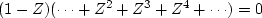.More formally, consider output Y(Z)=(1-Z)X(Z) made from the filter (1-Z) and any input X(Z). Since (1-Z) vanishes at Z=1, then likewise Y(Z) must vanish at Z=1. Vanishing at Z=1 is vanishing at frequency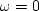because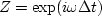from (20). Now we can recognize that multiplication of two functions of Z or ofis the equivalent of convolving the associated time functions.

 Multiplication in the frequency domain is convolution in the time domain.

A popular mathematical abbreviation for the convolution operator is an asterisk: equation (9), for example, could be denoted by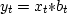.I do not disagree with asterisk notation, but I prefer the equivalent expression Y(Z)=X(Z)B(Z), which simultaneously exhibits the time domain and the frequency domain.

The filter (1-Z) is often called a differentiator.'' It is displayed in Figure 7.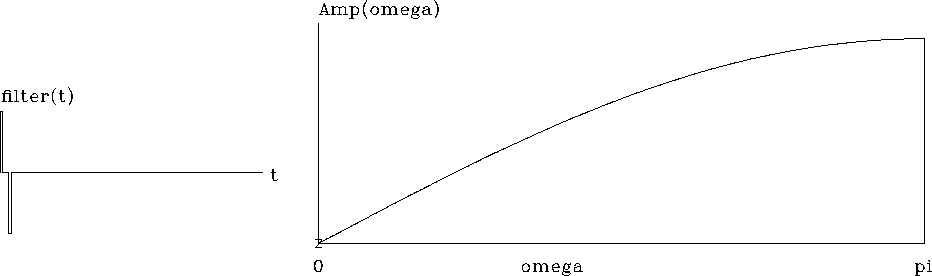ddt
Figure 7
A discrete representation of the first-derivative operator. The filter (1,-1) is plotted on the left, and on the right is an amplitude response, i.e., |1-Z| versus. (Press button to activate program Zplane. See appendix for details.)

The letter z'' plotted at the origin in Figure 7 denotes the root of 1-Z at Z=1, where.Another interesting filter is 1+Z, which destroys the highest possible frequency,where.

A root is a numerical value for which a polynomial vanishes. For example,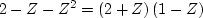vanishes whenever Z=-2 or Z=1. Such a root is also called a zero.'' The fundamental theorem of algebra says that if the highest power of Z in a polynomial is ZN, then the polynomial has exactly N roots, not necessarily distinct. As N gets large, finding these roots requires a sophisticated computer program. Another complication is that complex numbers can arise. We will soon see that complex roots are exactly what we need to design filters that destroy any frequency.Next: Gaussian examples Up: FOURIER AND Z-TRANSFORM Previous: Unit circle
Stanford Exploration Project
10/21/1998